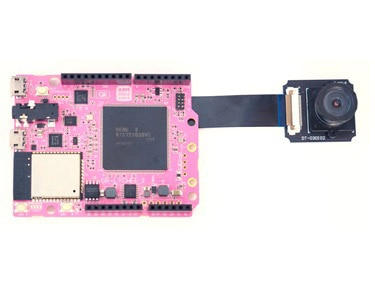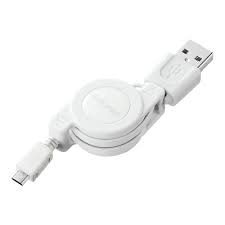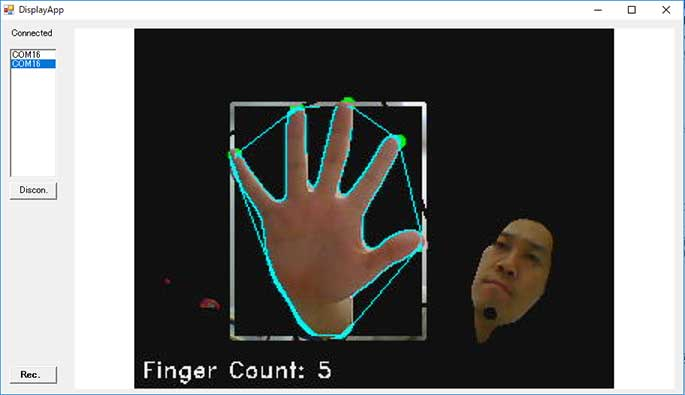## Overview

As an application example of OpenCV, this time we will run Ahmet Yaylalioglu's example of "Counting Fingers" with GR-LYCHEE. This is an example for an application using detection of contours, unevenness and skin color detection using HSV color space, etc.

Since the Renesas RZ/A1LU MPU mounted on the GR-LYCHEE board has 3MB of RAM, what can be processed is limited. Also, the memory to be written to the ROM also becomes large, and the write time becomes long to about 30 seconds, please be aware of this.

## Preparation

### Hardware

Prepare the GR-LYCHEE board and a USB cable (Micro B type). For details on how to install the camera, refer to the How to Attach the Camera, Accessories, LCD project.

### Software

Download and unzip the DisplayApp file. It is an application for checking the camera image with a PC.## Count Fingers

Execute the following program.

Since programming takes about 30 seconds, please do not disconnect the USB cable until the Mbed drive is recognized again after writing is complete.

``````
// GR-LYCHEE OpenCV finger count example.
// Referred :
// https://github.com/redbeardanil/Estimation-of-the-number-of-real-time-hand-fingers-with-image-processing/blob/master/ElAlgilaV2.cpp

#include <Arduino.h>
#include <Camera.h>
#include <DisplayApp.h>
#include <opencv.hpp>

using namespace cv;
#define IMAGE_HW 320
#define IMAGE_VW 240
// 1MB of NC_BSS is not used for malloc function.
// It's better to secure buffer as a static memory in this NC_BSS
uint8_t bgr_buf[3 * IMAGE_HW * IMAGE_VW]__attribute((section("NC_BSS"),aligned(32)));
uint8_t hsv_buf[3 * IMAGE_HW * IMAGE_VW]__attribute((section("NC_BSS"),aligned(32)));
uint8_t syn_buf[3 * IMAGE_HW * IMAGE_VW]__attribute((section("NC_BSS"),aligned(32)));

Camera camera(IMAGE_HW, IMAGE_VW);
DisplayApp display_app;
bool g_mode = false;

void setup() {
camera.begin();
delay(100);
}

void loop() {
static unsigned long last_time = 0;
if((millis() - last_time) > 2000){
g_mode = !g_mode;
last_time = millis();
}

Mat img_bgr(IMAGE_VW, IMAGE_HW, CV_8UC3, bgr_buf);
cvtColor(img_raw, img_bgr, COLOR_YUV2BGR_YUYV); // copy camera image to img_bgr

Mat img_hsv(IMAGE_VW, IMAGE_HW, CV_8UC3, hsv_buf);
cvtColor(img_bgr, img_hsv, COLOR_BGR2HSV);

inRange(img_hsv, Scalar(0, 50, 50), Scalar(20, 255, 255), img_temp);
inRange(img_hsv, Scalar(160, 50, 50), Scalar(180, 255, 255), img_mask);

// mask processing for drawing a picture
Mat img_synthesis(IMAGE_VW, IMAGE_HW, CV_8UC3, syn_buf);
img_synthesis = Scalar(0, 0, 0);

// find contours
vector<vector<Point> > contours;
vector<Vec4i> hierarchy;

int fingerCount = 0;
if (contours.size() > 0){
size_t indexOfBiggestContour = -1;
size_t sizeOfBiggestContour = 0;
for (size_t i = 0; i < contours.size(); i++){
if (contours[i].size() > sizeOfBiggestContour){
sizeOfBiggestContour = contours[i].size();
indexOfBiggestContour = i;
}
}
vector<vector<int> >hull(contours.size());
vector<vector<Point> >hullPoint(contours.size()); //elin hareketine göre eli çevreleyen çokgen
vector<vector<Vec4i> > defects(contours.size()); //parmak uclarindaki yesil noktalar..multi dimensional matrix
vector<vector<Point> >defectPoint(contours.size()); //point olarak parmak ucu noktalarýný x,y olarak tutuyor
vector<vector<Point> >contours_poly(contours.size()); //eli çevreleyen hareketli dikdörtgen
Point2f rect_point;
vector<RotatedRect>minRect(contours.size());
vector<Rect> boundRect(contours.size());
for (size_t i = 0; i<contours.size(); i++){
if (contourArea(contours[i])>5000){
convexHull(contours[i], hull[i], true);
convexityDefects(contours[i], hull[i], defects[i]);
if (indexOfBiggestContour == i){
minRect[i] = minAreaRect(contours[i]);
for (size_t k = 0; k<hull[i].size(); k++){
int ind = hull[i][k];
hullPoint[i].push_back(contours[i][ind]);
}
for (size_t k = 0; k<defects[i].size(); k++){
if (defects[i][k]>13 * 256){
int p_start = defects[i][k];
int p_end = defects[i][k];
int p_far = defects[i][k];
defectPoint[i].push_back(contours[i][p_far]);
circle(img_synthesis, contours[i][p_end], 3, Scalar(0, 255, 0), 2); //i ydi
fingerCount++;
}

}

drawContours(img_synthesis, contours, i, Scalar(255, 255, 0), 2, 8, vector<Vec4i>(), 0, Point());
drawContours(img_synthesis, hullPoint, i, Scalar(255, 255, 0), 1, 8, vector<Vec4i>(), 0, Point());
drawContours(img_mask, hullPoint, i, Scalar(0, 0, 255), 2, 8, vector<Vec4i>(), 0, Point());
approxPolyDP(contours[i], contours_poly[i], 3, false);
boundRect[i] = boundingRect(contours_poly[i]);
rectangle(img_mask, boundRect[i].tl(), boundRect[i].br(), Scalar(255, 0, 0), 2, 8, 0);
minRect[i].points(rect_point);
for (size_t k = 0; k<4; k++){
line(img_mask, rect_point[k], rect_point[(k + 1) % 4], Scalar(0, 255, 0), 2, 8);
}
}
}
}
}

if(g_mode){ img_mask = Scalar(255); }// for showing original image

// draw text
stringstream ss;
ss << "Finger Count: " << fingerCount;
putText(img_synthesis, ss.str(), Point(5, img_synthesis.rows - 8),
FONT_HERSHEY_DUPLEX, 0.5, Scalar(255, 255, 255), 1);

// display image
size_t jpegSize = camera.createJpeg(IMAGE_HW, IMAGE_VW, img_synthesis.data, Camera::FORMAT_RGB888);

}
``````

Connect the USB cable to USB0 on GR-LYCHEE and check the image with DisplayApp. Every 2 seconds, the original image and the image processing are switched. You can see how finger count is detected by skin color detection using HSV color space, contour detection, and convex Hull, convexityDefects.## About Using 1MB of Non-Cache Memory

Sections of the GR-LYCHEE RAM (3MB) are separated as shown in the following table. The L_TTB area 16KB to which the MMU table is allocated, the RAM area with cache 1.875MB, the RAM_NC area without cache 1MB.

When defining variables and arrays, RAM_NC area must explicitly specify __attribute ((section ("NC_BSS"), aligned (32))).